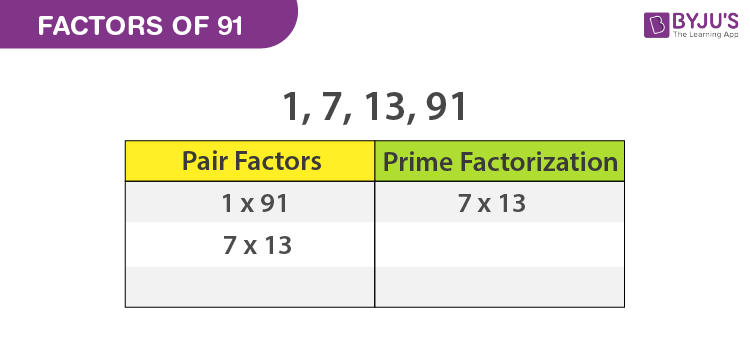# Factors of 91

Factors of 91 are the numbers that divide the original number completely or evenly. The two numbers that result in 91, after multiplication, are the pair factors of 91.

The factors of real number, 91,  are 1, 7 13, and 91They are all integers and not fractions. To find the factors of a number, 91, we can use the factorization method.In the factorization method, first take the numbers, 1 and 91 as factors and continue with finding the other pair of multiples of 91 which gives the results as an original number. To understand this method in a better way, read the article below to find the factors of 91 in pairs. Also, the prime factors of 91 with the help of the division method is discussed here

## Pair Factors of 91

To find the pair factors, multiply the two numbers in a pair to get the original number as 91, such numbers are as follows

 Pair Factors 1 × 91 = 91 ⇒ (1, 91) 7 × 13 = 91 ⇒ (7, 13)

Therefore, the pair factors are (1,91) and (7, 13).

Similarly, we can write negative pair factors. If we multiply two negative factors, then we get the original number, which is positive.

(-1) × (-91) = 91

(-7) × (-13) = 91

Therefore, the negative pair factors are (-1, -91) and (-7, -13).

## How to calculate the Factors of 91?

Learn the following steps to calculate the factors of a number 91.

• First, write the number 91
• Find the two numbers, which give the result as 91 under the multiplication, say 7 and 13, such as 7 × 13 = 91.
• We know that 7 and 13 are prime numbers that each number has only two factors, i.e., 1 and the number itself. So, it cannot be further factorized.

7 = 1 × 7

13 = 1 x 13

• Therefore, the factorization of 91 is written as 91 = 7 x 13 x 1
• Finally, write down all the unique numbers which we can obtain from 7 x 13 x 1
 Factors of 91 1, 7, 13, and 91

## Prime Factors of 91

The number 91 is a composite and it should have prime factors. Now let us know how to calculate the prime factors.

• The first step is to divide the number 91 with the smallest prime factor, say 7.

91 ÷ 7 = 13

• When you divide 13 by 7, you will get a fraction, that cannot be a factor of a number. So proceed with the next prime factor, say 13

13 ÷ 13 = 1

Finally, we received the number 1 at the end of the division process. So that we cannot proceed further. So, the prime factors are written as 7 x 13, where 7 and 13 are the prime numbers.

## Video Lesson on Prime Factors## Solved Examples

Q.1: If there are 13 members in a hall and each of them has 7 books, then how many total books are there?

Solution: Given,

Number of members = 13

Number of books each member has = 7

Total number of books = 13 x 7 = 91

Q.2: What are the common factors of 91 and 126?

Solution: We know that the factors of 91 are 1, 7, 13, 91.

Factors of 126 = 1, 2, 3, 6, 7, 9, 14, 18, 21, 42, 63, 126

Therefore, 1 and 7 are the only common factors of 91 and 126.

Register with BYJU’S – The Learning App to learn the factors of 91 and also the factors and prime factors of other numbers by factorization and division method.

## Frequently Asked Questions – FAQs

Q1

### What are the factors of 91?

The factors of 91 are 1, 7, 13 and 91. So basically, apart from 1 and 91 itself, 7 and 13 are the only factors.
Q2

### Are the multiples and factors of 91 the same?

Multiples and factors are two different concepts in Mathematics, both are not the same.
Q3

### What are the multiples of 91 in Maths?

The multiples of 91 are 91, 182, 273, 364, 455, 546, 637, 728, 819 and 910.
Q4

### Which is the second greatest number factor of 91?

The second greatest factor of 91 is 13. 13 divided 91 into 7 equal parts.
Q5

### Which is the greatest prime factor of 91?

The greatest prime factor of 91 is 13.
 Links Related to Factors Factors of 8 Factor of 36 Factors of 48 Factors of 18 Factors of 24 Factors of 25 Factors of 42 Factors of 60 Factors of 35 Factors of 81 Factors of 75 Factors of 56
Test your knowledge on Factors of 91